Author: Oscar Cronquist Article last updated on October 13, 2021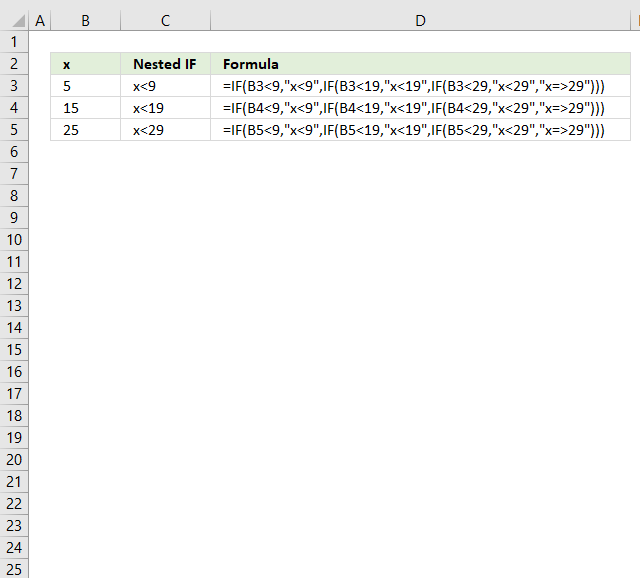Nested IF statements in a formula are multiple combined IF functions so more conditions and outcomes become possible. They all are more or less complicated to read and thankfully there are better alternatives.

You are allowed to use up to 254 nested IF statements but before you do that make sure you read this article, I promise you it will save you a lot of time.

This article will provide step-by-step tutorials for your specific scenario. If you can't find what you are looking for here please comment and I will add the missing part.

## 1. How to simplify nested IF functions based on a numerical range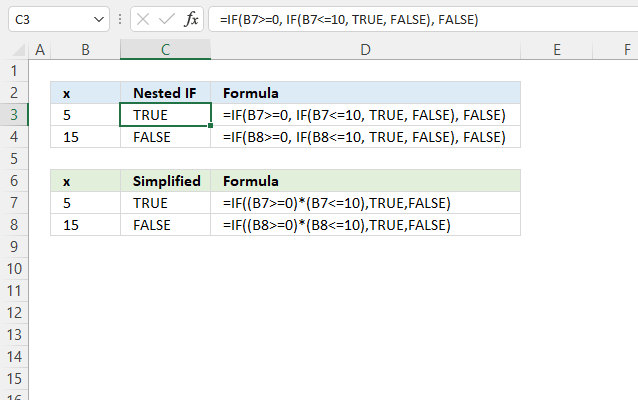You don't need to use multiple IF statements if you want to check if a cell value is in a given numerical range, it is enough to simply use two logical expressions in the first argument.

Nested IF function formula in cell C3:

=IF(B3>=0, IF(B3<=10, TRUE, FALSE), FALSE)

The formula above demonstrates a nested IF function. The following formula simplifies the formula above, it returns TRUE if the value in cell B3 is equal to or greater than 0 and is equal to or smaller than 10.

Formula in cell C7:

=IF((B3>=0)*(B3<=10), TRUE, FALSE)

Each logical expression is encapsulated with parentheses, this makes sure that the comparisons are made before multiplying the expressions. In other words, the parentheses determine the order of calculation.

The asterisk between the logical expressions means that both logical expressions must return TRUE for the logical test argument to return TRUE.

### 1.1 Explaining formula in cell C7

The formula in cell C7 is easier to understand and troubleshoot than the formula in cell C7.

#### Step 1 - First logical expression

The larger than character and equal sign are logical operators, they let you compare values and returns boolean values True or False.

B3>=0

becomes

5>=0

and returns boolean value True.

#### Step 2 - Second logical expression

B3<=10

becomes

5<=10

and returns boolean value True.

#### Step 3 - Multiply logical expressions - AND logic

We must check that both conditions are met and to do that we use the asterisk to multiply the expressions.

Use parentheses to control the order of calculations, we need to compare values before we multiply.

(B3>=0)*(B3<=10)

becomes

True * True

and returns 1. 1 is the numerical equivalent to True and False is 0 (zero).

AND logic returns True only if both conditions are met.

TRUE * TRUE = TRUE (1)
TRUE * FALSE= FALSE(0)
FALSE* TRUE = FALSE(0)
FALSE* FALSE= FALSE(0)

#### Step 4 - Evaluate IF function

The IF function returns one value if the logical test evaluates to TRUE and another value if the logical test returns FALSE.

IF(logical_test, [value_if_true], [value_if_false])

IF((B3>=0)*(B3<=10), TRUE, FALSE)

becomes

IF(1, TRUE, FALSE)

and returns TRUE.

## 2. How to simplify nested IF statements based on numerical ranges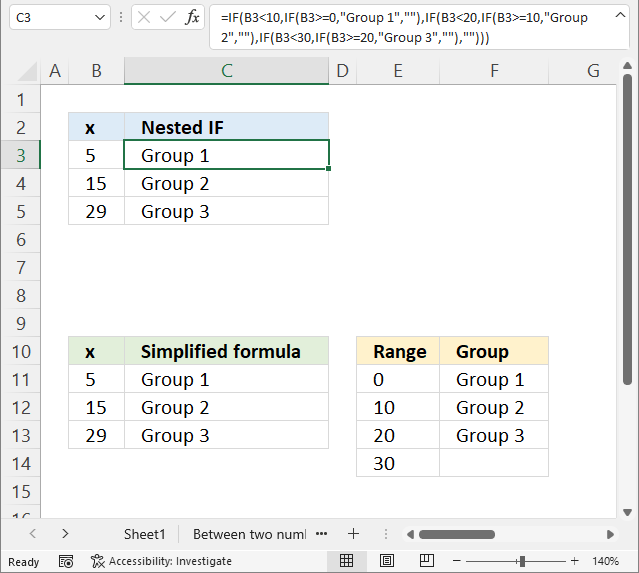The picture above shows you nested IF statements that allow you to return different outcomes depending on the value in column B.

Nested If functions formula in cell C3:

=IF(B3<10, IF(B3>=0, "Group 1", ""), IF(B3<20, IF(B3>=10, "Group 2", ""), IF(B3<30, IF(B3>=20, "Group 3", ""), "")))

A value equal to or greater than 0 and smaller than 10 is in "Group 1".
A value equal to or greater than 10 and smaller than 20 returns "Group 2".
A value equal to or greater than 20 and smaller than 30 returns "Group 3".

The simplified formula in cell C11:

=VLOOKUP(B11, \$E\$11:\$F\$14, 2, TRUE)

This formula is considerably smaller and doesn't grow bigger if you need more criteria.

### 2.1 Explaining formula in cell C11

#### Step 1 - Build the table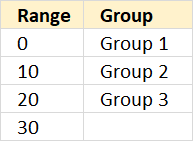The table above defines the numerical ranges and what value to return. Note, that there are no gaps between the ranges.

#### Step 2 - VLOOKUP function

The VLOOKUP function lets you search the leftmost column for a value and return another value on the same row in a column you specify.

VLOOKUP(lookup_value, table_array, col_index_num, [range_lookup])

lookup_value - A value.
table_array -
The range you want to use, remember that the VLOOKUP function always looks in the leftmost column in your specified range.
col_index_num -
The column number which contains the return value.
[range_lookup] -
True or False. True - approximate match, the leftmost column must be in ascending order. False - Exact match.

#### Step 3 - Populate arguments

VLOOKUP(lookup_value, table_array, col_index_num, [range_lookup])

lookup_value - B11
table_array - \$E\$11:\$F\$14

col_index_num -
2
[range_lookup] -
True.

VLOOKUP(lookup_value, table_array, col_index_num, [range_lookup])

becomes

VLOOKUP(B11, \$E\$11:\$F\$14, 2, TRUE)

#### Step 4 - Evaluate VLOOKUP function

VLOOKUP(B11, \$E\$11:\$F\$14, 2, TRUE)

becomes

VLOOKUP(5, {0, "Group 1"; 10, "Group 2"; 20, "Group 3"; 30, 0}, 2, TRUE)

and returns "Group 1" in cell C11.The last argument TRUE lets you perform an approximate match meaning it matches an item equal to or next smaller item if no exact match is found. This is why it is so important to have the table sorted in ascending order.

Value 5 matches no value in the table, however, it is between 0 (zero) and 10. The next smaller value is 0 (zero), the corresponding value in column F on the same row is "Group 1".

### 2.2 How to add criteria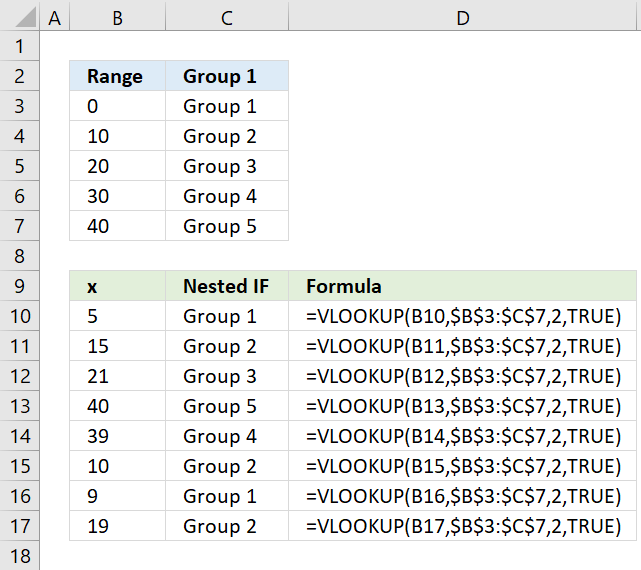Imagine that you have 5 different groups, you now need to add six more nested IF statements. The formula grows considerably, however, the VLOOKUP function is really useful in this case.

The VLOOKUP lets you easily group numbers using a simple function instead of constructing a mega formula that is hard to follow and troubleshoot.

Need more groups? No, problem. Add groups to the first table and then adjust the cell reference in the second argument in the VLOOKUP function.

=VLOOKUP(B10, \$B\$3:\$C\$7, 2, TRUE)

Note, the first table must have the first column sorted from small to large. Make sure you use TRUE in the fourth VLOOKUP argument. This means that it only needs an approximate match.

## 3. How to simplify nested IF functions based on conditions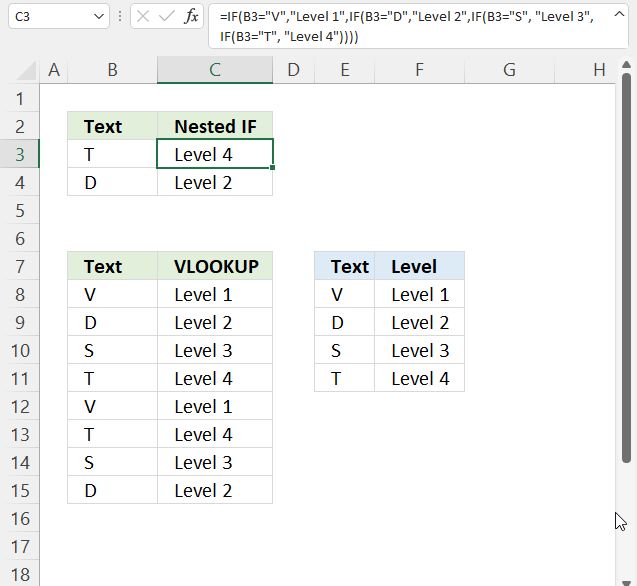These nested IF statements in cell C3 check if a value is equal to a condition and returns another value if True.

Nested IF function formula in cell C3:

=IF(B3="V", "Level 1", IF(B3="D", "Level 2", IF(B3="S", "Level 3", IF(B3="T", "Level 4"))))

You can use the VLOOKUP function in this case as well as an alternative to nested IF functions. You need to find an exact match in this case so change the fourth VLOOKUP argument to FALSE.

The simplified formula in cell C8:

=VLOOKUP(B8, \$E\$8:\$F\$11, 2, FALSE)

The VLOOKUP function looks for the value in the first column (E8:F11) and returns the corresponding value in the second column. That is why I use 2 in the third VLOOKUP argument.

### 3.1 Explaining formula in cell C11

#### Step 1 - Build table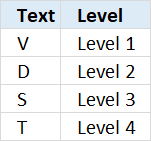The table above defines the conditions and what value to return.

#### Step 2 - VLOOKUP function

The VLOOKUP function lets you search the leftmost column for a value and return another value on the same row in a column you specify.

VLOOKUP(lookup_value, table_array, col_index_num, [range_lookup])

lookup_value - A value.
table_array -
The range you want to use, remember that the VLOOKUP function always looks in the leftmost column in your specified range.
col_index_num -
The column number which contains the return value.
[range_lookup] -
True or False. True - approximate match, the leftmost column must be in ascending order. False - Exact match.

#### Step 3 - Populate arguments

VLOOKUP(lookup_value, table_array, col_index_num, [range_lookup])

lookup_value - B8
table_array - \$E\$8:\$F\$11

col_index_num -
2
[range_lookup] -
FALSE.

VLOOKUP(lookup_value, table_array, col_index_num, [range_lookup])

becomes

VLOOKUP(B8, \$E\$8:\$F\$11, 2, TRUE)

#### Step 4 - Evaluate VLOOKUP function

VLOOKUP(B8, \$E\$8:\$F\$11, 2, TRUE)

becomes

VLOOKUP("V", {"V", "Level 1";"D", "Level 2";"S", "Level 3";"T", "Level 4"}, 2, TRUE)

and returns "Level 1" in cell C8.Recommended articles

Use VLOOKUP to calculate discounts, commissions, tariffs, charges, shipping costs, packaging expenses or bonuses
Have you ever tried to build a formula to calculate discounts based on price? The VLOOKUP function is much easier […]

## 4. How to simplify nested IF functions based on date ranges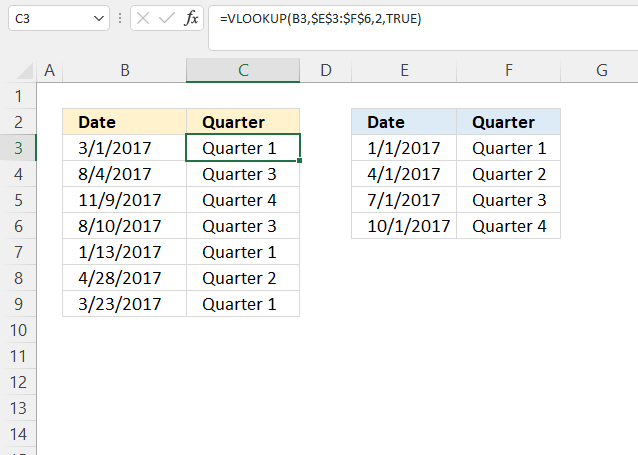The above image demonstrates how to use multiple date ranges with a short and simple VLOOKUP function. A date range consists of two dates, since these date ranges are contiguous the end date also represents the start date for the next range.

Formula in cell C3:

=VLOOKUP(B3, \$E\$3:\$F\$6, 2, TRUE)

The first cell range is between 1/1/2017 and 2/15/2017. The second cell range is between 3/1/2017 and 6/1/2017.

### 4.1 Explaining formula in cell C11

Excel dates are really not much different from numerical values, in fact, they are numerical values formatted as dates.

1 is 1/1/1900 and 1/1/2000 is 36526. We can use the same technique described in section 2 to extract the correct quarter.

#### Step 1 - Build a table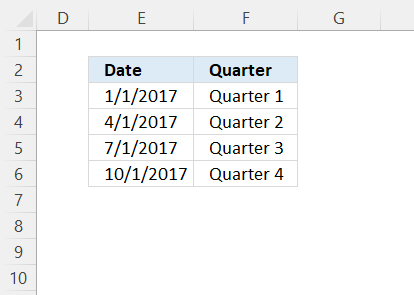The table above defines the date ranges and what value to return. Note, that there are no gaps between the date ranges.

#### Step 2 - VLOOKUP function

The VLOOKUP function lets you search the leftmost column for a value and return another value on the same row in a column you specify.

VLOOKUP(lookup_value, table_array, col_index_num, [range_lookup])

lookup_value - A value.
table_array -
The range you want to use, remember that the VLOOKUP function always looks in the leftmost column in your specified range.
col_index_num -
The column number which contains the return value.
[range_lookup] -
True or False. True - approximate match, the leftmost column must be in ascending order. False - Exact match.

#### Step 3 - Populate arguments

VLOOKUP(lookup_value, table_array, col_index_num, [range_lookup])

lookup_value - B3
table_array - \$E\$3:\$F\$6

col_index_num -
2
[range_lookup] -
True.

VLOOKUP(lookup_value, table_array, col_index_num, [range_lookup])

becomes

VLOOKUP(B3, \$E\$3:\$F\$6, 2, TRUE)

#### Step 4 - Evaluate VLOOKUP function

VLOOKUP(B3, \$E\$3:\$F\$6, 2, TRUE)

becomes

=VLOOKUP(42795, {42736, "Quarter 1"; 42826, "Quarter 2"; 42917, "Quarter 3"; 43009, "Quarter 4"}, 2, TRUE)

and returns "Group 1" in cell C3.